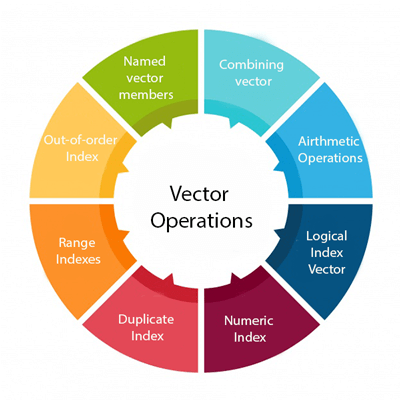# Vector operations in R

## Vector Operation

In R, there are various operation which is performed on the vector. We can add, subtract, multiply or divide two or more vectors from each other. In data science, R plays an important role, and operations are required for data manipulation. There are the following types of operation which are performed on the vector.### 1) Combining vectors

The c() function is not only used to create a vector, but also it is also used to combine two vectors. By combining one or more vectors, it forms a new vector which contains all the elements of each vector. Let see an example to see how c() function combines the vectors.

Example:

``````p<-c(1,2,4,5,7,8)
q<-c("shubham","arpita","nishka","gunjan","vaishali","sumit")
r<-c(p,q)
``````

Output

`````` "1"        "2"        "4"        "5"        "7"        "8"
 "shubham"  "arpita"   "nishka"   "gunjan"   "vaishali" "summit"
``````

### 2) Arithmetic operations

We can perform all the arithmetic operation on vectors. The arithmetic operations are performed member-by-member on vectors. We can add, subtract, multiply, or divide two vectors. Let see an example to understand how arithmetic operations are performed on vectors.

Example:

a<-c(1,3,5,7)
b<-c(2,4,6,8)
a+b
a-b
a/b
a%%b

Output

``````  3  7 11 15
 -1 -1 -1 -1
  2 12 30 56
 0.5000000 0.7500000 0.8333333 0.8750000
 1 3 5 7
``````

### 3) Logical Index vector

With the help of the logical index vector in R, we can form a new vector from a given vector. This vector has the same length as the original vector. The vector members are TRUE only when the corresponding members of the original vector are included in the slice; otherwise, it will be false. Let see an example to understand how a new vector is formed with the help of logical index vector.

Example:

a<-c(“Shubham”,“Arpita”,“Nishka”,“Vaishali”,“Sumit”,“Gunjan”)
b<-c(TRUE,FALSE,TRUE,TRUE,FALSE,FALSE)
a[b]

Output

 “Shubham” “Nishka” “Vaishali”

### 4) Numeric Index

In R, we specify the index between square braces [ ] for indexing a numerical value. If our index is negative, it will return us all the values except for the index which we have specified. For example, specifying [-3] will prompt R to convert -3 into its absolute value and then search for the value which occupies that index.

Example:

``````q<-c("shubham","arpita","nishka","gunjan","vaishali","sumit")
q
q[-4]
q
``````

Output

`````` "arpita"
 "shubham"  "arpita"   "nishka"   "vaishali" "sumit"
 NA
``````

### 5) Duplicate Index

An index vector allows duplicate values which means we can access one element twice in one operation. Let see an example to understand how duplicate index works.

Example:

q<-c(“shubham”,“arpita”,“nishka”,“gunjan”,“vaishali”,“sumit”)
q[c(2,4,4,3)]

Output

 “arpita” “gunjan” “gunjan” “nishka”

### 6) Range Indexes

Range index is used to slice our vector to form a new vector. For slicing, we used colon(operator. Range indexes are very helpful for the situation involving a large operator. Let see an example to understand how slicing is done with the help of the colon operator to form a new vector.

Example:

q<-c(“shubham”,“arpita”,“nishka”,“gunjan”,“vaishali”,“sumit”)
b<-q[2:5]
b

Output

 “arpita” “nishka” “gunjan” “vaishali”

### 7) Out-of-order Indexes

In R, the index vector can be out-of-order. Below is an example in which a vector slice with the order of first and second values reversed.

Example:

``````q<-c("shubham","arpita","nishka","gunjan","vaishali","sumit")b<-q[2:5]
q[c(2,1,3,4,5,6)]
``````

Output

 “arpita” “shubham” “nishka” “gunjan” “vaishali” “summit”

### 8) Named vectors members

We first create our vector of characters as:

z=c(“TensorFlow”,“PyTorch”)
z

Output

 “TensorFlow” “PyTorch”
Once our vector of characters is created, we name the first vector member as “Start” and the second member as “End” as:

names(z)=c(“Start”,“End”)
z

Output

``````Start              End
"TensorFlow"    "PyTorch"
``````

We retrieve the first member by its name as follows:

z[“Start”]

Output

Start
“TensorFlow”

We can reverse the order with the help of the character string index vector.

z[c(“Second”,“First”)]

Output

`````` Second        First
"PyTorch"    "TensorFlow"
``````

## Applications of vectors

1. In machine learning for principal component analysis vectors are used. They are extended to eigenvalues and eigenvector and then used for performing decomposition in vector spaces.
2. The inputs which are provided to the deep learning model are in the form of vectors. These vectors consist of standardized data which is supplied to the input layer of the neural network.
3. In the development of support vector machine algorithms, vectors are used.
4. Vector operations are utilized in neural networks for various operations like image recognition and text processing.